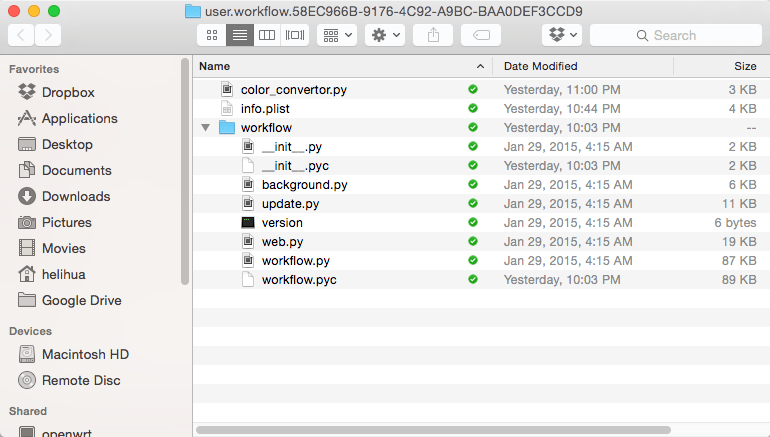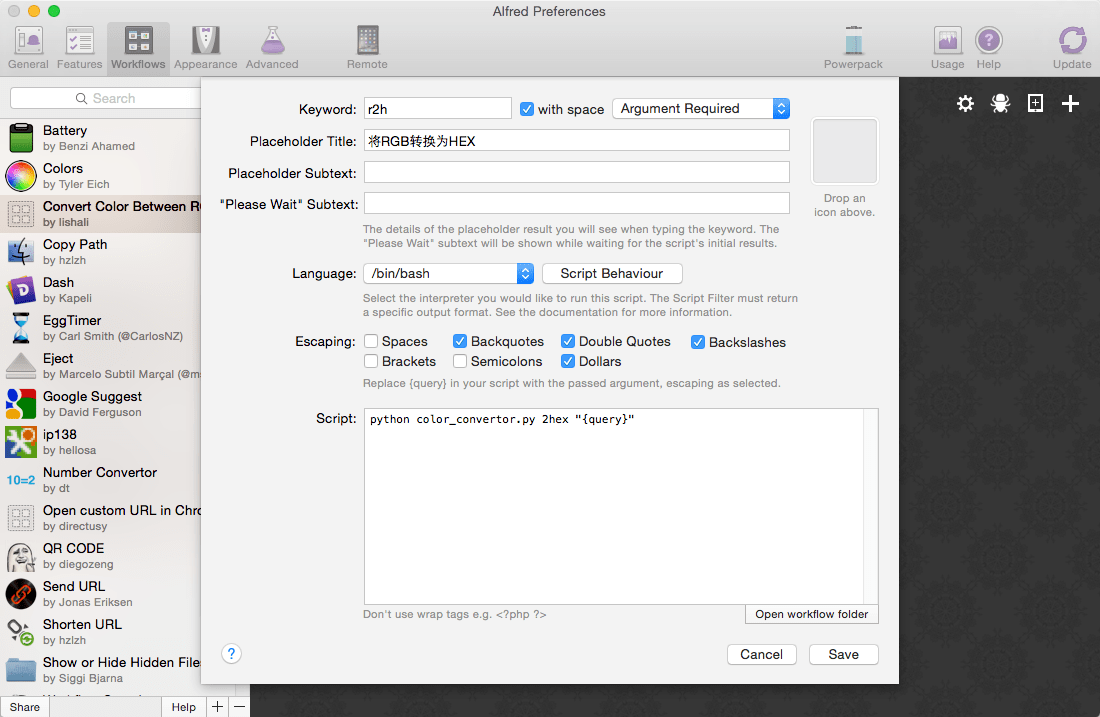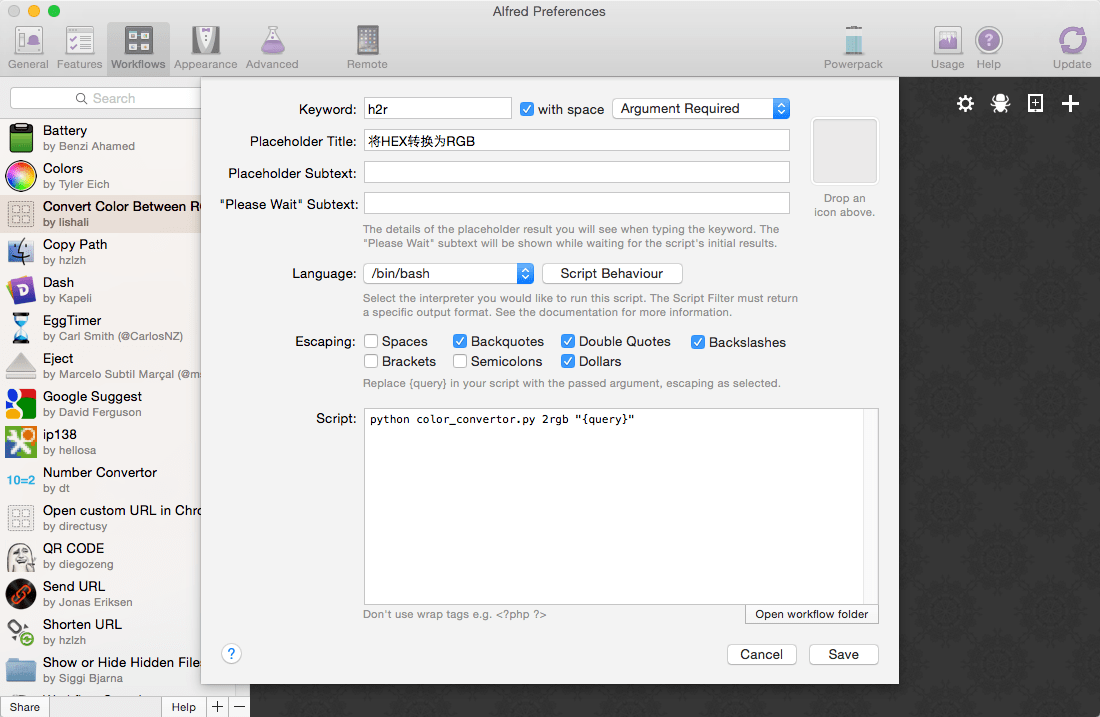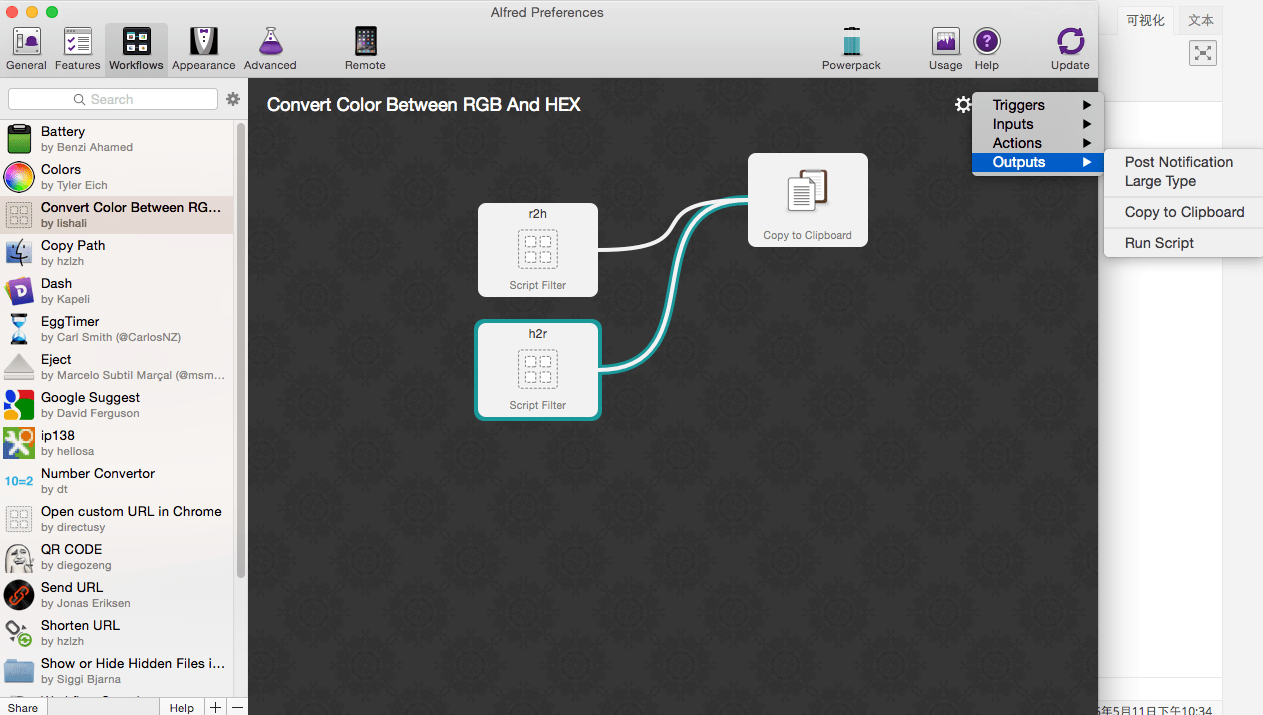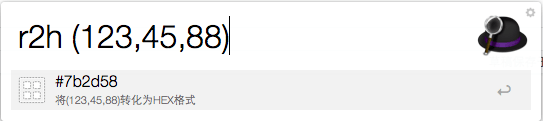# 用 Python 写一个简单的 Alfred Workflow 用于颜色值的转换

Alfred 是我使用 Mac 之后安装的第二个工具软件（正常工作需要的开发软件和浏览器之类的通用软件不算），第一个是 Dash，第二个是 Alfred。Dash 是在试用了 5 分钟不到，查看 Android 帮助文档如飞的响应速度，让我直接购买了正版授权。Alfred 是在当做 Launch 软件使用了快 1 年之后，看了很多人提到的关于 Workflow 的增强功能之后，在某个非常想购物的日子里头买了一个 PowerPack，从此用上了 Workflow 的功能，当然在这一年里头实际上我也就只用了有道词典这么一个 Workflow，其他的几乎不怎么使用，主要还是习惯问题。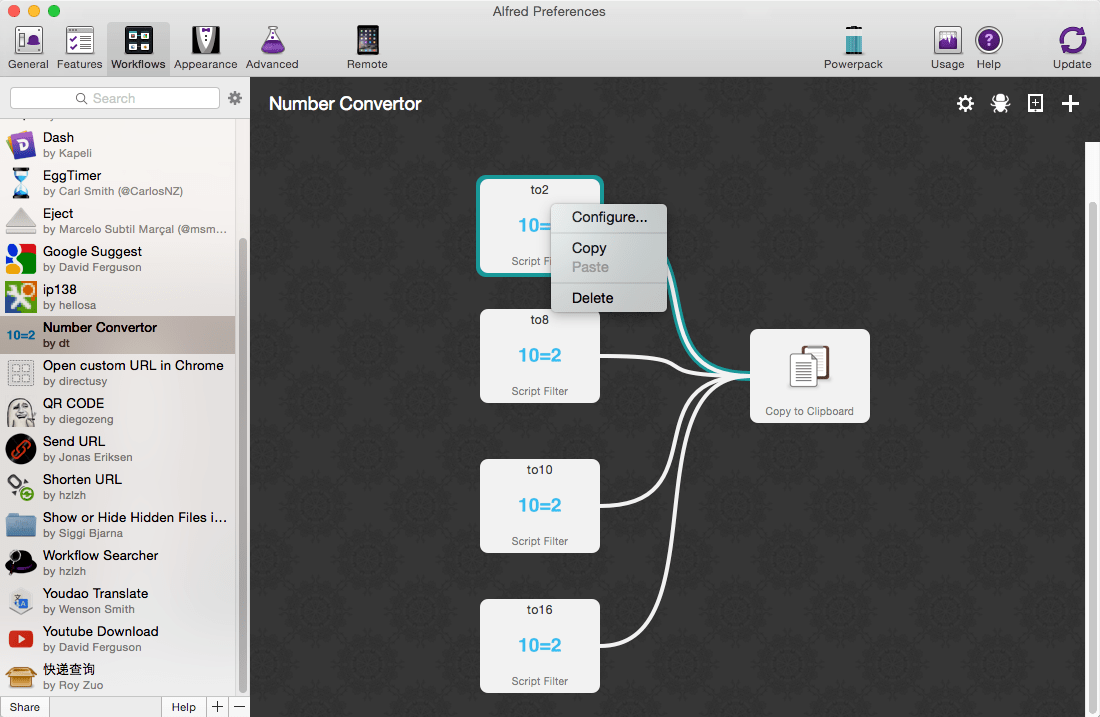```# -*- coding: utf-8 -*-
import sys
from workflow import Workflow

def main(wf):
cmd = wf.args
input_str = wf.args

values_str = ""
if cmd == "2rgb":
values_str = conver_hex_to_rgb(input_str)
desc = u'将%s 转化为 RGB 格式' % (input_str)
elif cmd == "2hex":
values_str = convert_rgb_to_hex(input_str)
desc = u'将%s 转化为 HEX 格式' % (input_str)
wf.send_feedback()

def ignore_exception(IgnoreException=Exception,DefaultVal=None):
""" Decorator for ignoring exception from a function
e.g.   @ignore_exception(DivideByZero)
e.g.2. ignore_exception(DivideByZero)(Divide)(2/0)
"""
def dec(function):
def _dec(*args, **kwargs):
try:
return function(*args, **kwargs)
except IgnoreException:
return DefaultVal
return _dec
return dec

sint = ignore_exception(ValueError,0)(int)

def convert_decimal_to_hex(numstr):
decimal_var = sint(numstr)
return "{0:#0{1}x}".format(decimal_var,4)[2:]

def convert_rgb_to_hex(rgb_str):
if rgb_str.startswith("(") and rgb_str.endswith(")"):
splits = rgb_str.strip("()").split(",")
if (len(splits) == 3):
red_decimal_str = splits
red_hex = convert_decimal_to_hex(red_decimal_str)
green_decimal_str = splits
green_hex = convert_decimal_to_hex(green_decimal_str)
blue_decimal_str = splits
blue_hex = convert_decimal_to_hex(blue_decimal_str)
hex_str = "#" + red_hex + green_hex + blue_hex
return hex_str
else:
return ""
else:
return ""

def conver_hex_to_rgb(hex_str):
if hex_str.startswith("#"):
hex_str = hex_str[1:]
if len(hex_str) == 6:
red_decimal_var = sint(hex_str[0:2],16)
green_decimal_var = sint(hex_str[2:4],16)
blue_decimal_var = sint(hex_str[4:6],16)
return "(%d,%d,%d)" % (red_decimal_var, green_decimal_var, blue_decimal_var)
elif len(hex_str) == 3:
red_decimal_var = sint(hex_str[0:1] + hex_str[0:1],16)
green_decimal_var = sint(hex_str[1:2] + hex_str[1:2],16)
blue_decimal_var = sint(hex_str[2:3] + hex_str[2:3],16)
return "(%d,%d,%d)" % (red_decimal_var, green_decimal_var, blue_decimal_var)
else:
return ""

if __name__ == u"__main__":
wf = Workflow()
sys.exit(wf.run(main))

```